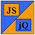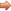# JavaScript & jQuery Tutorials

JS HomeJS Referencefunction

## function special operator

Functions are created using the global object constructor `aFunction = new Function()`,
via declaration using the `function` statement,
or by function expression using the `function` operator.

All functions are `Function` objects undernath the covers, but as mentioned above there are different ways to set up the function creation.

### Description

The `function` operator allows us to create a `Function` object using an expression. The function expression can have a name or be an anonymous function.

Function objects created this way are parsed with the rest of The JavaScript code and so are more efficient than functions created using the constructor creation method.

Below are examples of using the `function` operator.

``````
// Create a function operator that takes an argument and returns the square of the argument.
var squareResult = function(a) {
return a * a;
};
alert(squareResult(7) + ' Anonymous function expression');
alert(squareResult(15) + ' Anonymous function expression');
// Create a named function expression that takes an argument and returns the square of the argument.
var squareResult2 = function getSquare(a) {
return a * a;
};
alert(squareResult2(6) + ' Named function expression getSquare{}');
``````

Press the button below to action the above code:

## Related Tutorials

JavaScript Intermediate Tutorials - Lesson 8 - Functions